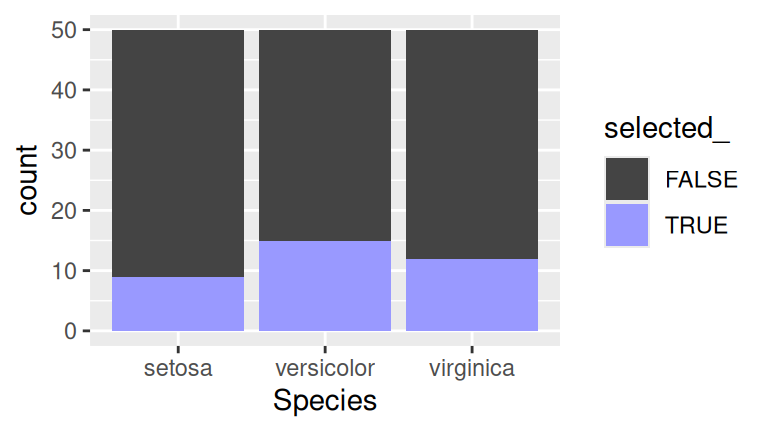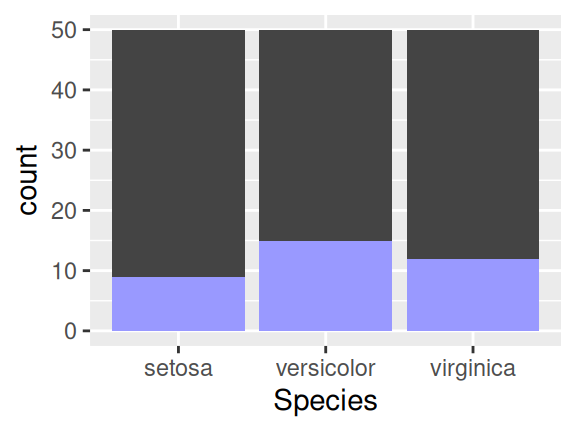Crosstalk doesn’t require the use of Shiny. You can perform linked brushing and filtering purely with JavaScript, leaving you with static HTML files that you can host on any web server.

On the other hand, you can use Crosstalk and Shiny together, and when you do so, you broaden the capabilities of Crosstalk by allowing your brushing and filtering to drive arbitrary calculations and Shiny outputs (and vice versa).

### Basic usage

We’ll start by creating a basic Shiny application that contains some linked widgets, simply so you can see how the parts fit together. If you’ve ever used HTML widgets with Shiny apps, this will look familiar. Our UI definition includes xxxOutput calls, which we then populate with server-side renderXXX expressions.

The main thing to note here is that the shared_iris variable that holds the SharedData instance is being declared inside the server function.

library(shiny)
library(crosstalk)
library(d3scatter)

ui <- fluidPage(
fluidRow(
column(6, d3scatterOutput("scatter1")),
column(6, d3scatterOutput("scatter2"))
)
)

server <- function(input, output, session) {
shared_iris <- SharedData$new(iris) output$scatter1 <- renderD3scatter({
d3scatter(shared_iris, ~Petal.Length, ~Petal.Width, ~Species, width = "100%")
})

output$scatter2 <- renderD3scatter({ d3scatter(shared_iris, ~Sepal.Length, ~Sepal.Width, ~Species, width = "100%") }) } shinyApp(ui, server) It’s important that the SharedData object be created inside the server function, not outside it. If you create SharedData outside of the server function, the bidirectional communication between Crosstalk’s client and server pieces will not work and some of the capabilities described below will fail to work. Run the above code, or click the Live Demo button just below it. This app works, but doesn’t do anything we couldn’t accomplish just as well without Shiny. Let’s see what else we can do. ### Using reactive data sources One thing you can’t do in a static Crosstalk HTML page is change your data (without regenerating and then reloading the page). With Shiny, it’s easy to set up reactive expressions that react to changes. Integrating reactive expressions with Crosstalk is easy. Take a reactive expression that produces a data frame, and simply pass the reactive expression into SharedData$new in place of a data frame.

In this example, we’ll modify the previous example on this page to randomly jitter the data a couple of times a second. (If random jittering is too contrived for your taste, imagine streaming data or interactively filtered data.)

library(shiny)
library(crosstalk)
library(d3scatter)

ui <- fluidPage(
fluidRow(
column(6, d3scatterOutput("scatter1")),
column(6, d3scatterOutput("scatter2"))
)
)

server <- function(input, output, session) {
jittered_iris <- reactive({
invalidateLater(500)
data.frame(lapply(iris, function(col) {
if (is.numeric(col))
jitter(col)
else
col
}))
})
shared_iris <- SharedData$new(jittered_iris) output$scatter1 <- renderD3scatter({
d3scatter(shared_iris, ~Petal.Length, ~Petal.Width, ~Species, width = "100%",
x_lim = range(iris$Petal.Length), y_lim = range(iris$Petal.Width))
})

output$scatter2 <- renderD3scatter({ d3scatter(shared_iris, ~Sepal.Length, ~Sepal.Width, ~Species, width = "100%", x_lim = range(iris$Sepal.Length), y_lim = range(iris$Sepal.Width)) }) } shinyApp(ui, server) Notice that we passed the reactive expression itself (jittered_iris), not the value of the reactive expression (jittered_iris()). We don’t want to read the reactive expression at the time that we construct the SharedData object, but instead, want to give the reactive expression itself to the new object so the latter can read the former at any point in the future. If this feels foreign to you, think of how you pass a function name, not a function call, to lapply; that’s exactly analogous to what we’re doing here. Note that when using a reactive expression for your underlying data source, the SharedData constructor’s key argument should be provided explicitly unless you know for sure that either 1) the data frame will always have row names, or 2) the keys of the rows you’re returning will never vary in order. If the former row 3 becomes the new row 15 while the app executes, and you have not specified keys, you’ll get strange results. And be sure not to provide the key argument as a character value, but instead as a formula or function (that will be applied against each new data frame produced by the reactive expression). ### Adding reactive outputs Not only can we use reactive data to feed SharedData and Crosstalk widgets (i.e. server-to-client); we can also use Crosstalk selections and filters to feed reactive expressions (i.e. client-to-server). We do this using the same SharedData object that we pass to the widgets. This object has a data() method that we can use to reactively read a snapshot of the data, and optionally, the selection state of all the rows. In this example we’ll use the Crosstalk selection to drive a textual summary of the selected data. (As a bonus, we’ll also let the user subset the underlying data frame by species, using a Shiny selectInput and a reactive. At the time of this writing, Crosstalk filter controls don’t work particularly well with Shiny, so you can use traditional Shiny techniques to do filtering instead.) library(shiny) library(crosstalk) library(d3scatter) library(dplyr) ui <- fluidPage( selectInput("species", "Species", levels(iris$Species), multiple = TRUE),
fluidRow(
column(6, d3scatterOutput("scatter1")),
column(6, d3scatterOutput("scatter2"))
),
h4("Summary of selected data"),
verbatimTextOutput("summary")
)

server <- function(input, output, session) {
# Subset the dataset based on user's choice of species
user_iris <- reactive({
iris[is.null(input$species) | iris$Species %in% input$species,] }) shared_iris <- SharedData$new(user_iris)

output$scatter1 <- renderD3scatter({ d3scatter(shared_iris, ~Petal.Length, ~Petal.Width, ~Species, width = "100%") }) output$scatter2 <- renderD3scatter({
d3scatter(shared_iris, ~Sepal.Length, ~Sepal.Width, ~Species, width = "100%")
})

output$summary <- renderPrint({ df <- shared_iris$data(withSelection = TRUE) %>%
filter(selected_ | is.na(selected_)) %>%
mutate(selected_ = NULL)

cat(nrow(df), "observation(s) selected\n\n")
summary(df)
})
}

shinyApp(ui, server)

Calling shared_iris$data() with the withSelection = TRUE argument gives us a data frame with an additional column selected_ that contains TRUE or FALSE (or NA for all the rows if there is no active selection). This is a reactive operation, so when the selection changes in the future, the output (or reactive expression, or observer) that called data(withSelection=TRUE) will automatically respond. ### Integrating with ggplot2 We can use the capabilities above to integrate Crosstalk widgets with interactive ggplot2 plots driven by Shiny. For those very familiar with ggplot2 and Shiny, reflecting selection state using fill or color is straightforward but getting nice looking results is a bit tedious. For example, this code is simple but the output has some problems: library(ggplot2) # Simulated sharedData$data(withSelection = TRUE)
df <- cbind(iris, selected_ = c(TRUE, FALSE, FALSE)[sample(3, nrow(iris), replace = TRUE)])

ggplot(df, aes(Species, fill = selected_)) +
geom_bar(stat = "count") +
scale_fill_manual(values = c("#444444", "#9999FF"))This code has several problems:

• The bars are highlighted from the top down, instead of from the bottom up. This can be fixed by converting the selection_ column to a factor with ordered levels.
• Though not seen in this example, when no selection is active the selection_ column is NA and must be converted to FALSE.
• The legend is superfluous when you have the realtime feedback of linked brushing.

Rather than forcing you to deal with these common problems each time, Crosstalk provides some helper functions: selection_factor(selected_) should be used in aes, and then scale_fill_selection or scale_color_selection should be used for scaling.

library(crosstalk)

ggplot(df, aes(Species, fill = selection_factor(selected_))) +
geom_bar(stat = "count") +
scale_fill_selection("#444444", "#9999FF")Much better!

The following code is a complete Shiny app that uses this approach. This example uses Shiny’s new debounce function, which filters a reactive expression to slow down its rate of change. Without it, too many updates come from Crosstalk and cause the ggplot2 code to appear laggy. (The debounce function has not, at time of this writing, been part of a CRAN release. You can install the latest development version of Shiny with devtools::install_github("rstudio/shiny").)

library(shiny)
library(crosstalk)
library(d3scatter)
library(ggplot2)

ui <- fluidPage(
fluidRow(
column(4, d3scatterOutput("scatter1")),
column(4, d3scatterOutput("scatter2")),
column(4, plotOutput("by_species"))
)
)

server <- function(input, output, session) {
shared_iris <- SharedData$new(iris) output$scatter1 <- renderD3scatter({
d3scatter(shared_iris, ~Petal.Length, ~Petal.Width, ~Species, width = "100%")
})

output$scatter2 <- renderD3scatter({ d3scatter(shared_iris, ~Sepal.Length, ~Sepal.Width, ~Species, width = "100%") }) df <- debounce(reactive(shared_iris$data(withSelection = TRUE)), 250)
output\$by_species <- renderPlot({
ggplot(df(), aes(Species, fill = crosstalk::selection_factor(selected_))) +
geom_bar(stat = "count") +
crosstalk::scale_fill_selection("#444444", "#9999FF")
})
}

shinyApp(ui, server)